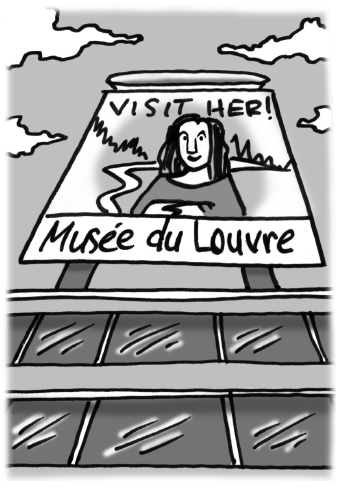### Home > INT2 > Chapter 10 > Lesson 10.1.1 > Problem10-11

10-11.

Multiple Choice: The Mona Lisa, by Leonardo da Vinci, is arguably the most famous painting in existence. The rectangular artwork, which hangs in the Musée du Louvre, measures $77$ cm by $53$ cm. When the museum created a billboard with an enlarged version of the portrait for advertisement, they used a linear scale factor of $20$. What is the area of the billboard?

The scale factor affects the individual measurements rather than the total area.1. $4081$ cm$^2$

1. $32{,}638{,}000$ cm$^2$

1. $81{,}620$ cm$^2$

1. $1{,}632{,}400$ cm$^2$

1. none of these